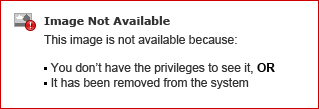ShowAll Questionssorted byDate PostedKumar G

# How to write a test class for user feed

I have got 58% code covereage for the chatterTooltip class mentioned in code sample and i am facing difficulty while increasing remaining code coverage.

```Class :

public class chatterTooltip {
public string uID   { get; set; }

public User u       { get; set; }

public integer followers    { get; set; }
public integer following    { get; set; }

public boolean followingme  { get; set; }

public string lastUpdate    { get; set; }
public string timestr       { get; set; }

public boolean isTest       { get; set; }

public chatterTooltip() {
isTest = false;
}

if( uID == null )
return null;

if( u != null )
return u.name;

User[] us = [select id, name
from User
where id = :uID];

if( us.isEmpty() )
return '';

u = us;

return u.name;
}

integer qlimit = ( isTest ? 1 : 1000 );

EntitySubscription[] ess = [select id, parentid
from EntitySubscription
where parentid = :uID
or subscriberid = :uID limit :qlimit];

followers = 0;

for( EntitySubscription es : ess )
if( es.parentid == uID )
followers++;

following = ess.size() - followers;

if( UserInfo.getUserId() != uID ) {
EntitySubscription[] ess2 = [select id
from EntitySubscription
where parentid = :UserInfo.getUserId()
and subscriberid = :uID];

followingme = ess2.size() > 0;
} else
followingme = false;

UserFeed[] ufs = [select id, FeedPost.body, createddate
from UserFeed
where parentid = :uID
order by createddate desc limit 1];

if( ufs.isEmpty() )
return;

lastUpdate = ufs.FeedPost.body;

if( lastUpdate == null )
return;

if( lastUpdate.length() > 70 )
lastUpdate = lastUpdate.substring( 0, 70 ) + '...';

timestr = relativeTime( ufs.createddate );
}

public string relativeTime( Datetime dt ) {
long diff =  ( Datetime.now().getTime() - dt.getTime() ) / 1000;
string unit;

if( diff < 60 )
unit = 'second';
else if( diff < 60 * 60 ) {
diff /= 60;
unit = 'minute';
} else if( diff < 60 * 60 * 24 ) {
diff = diff / 60 / 60;
unit = 'hour';
} else {
diff = diff / 60 / 60 / 24;
unit = 'day';
}

if( diff > 1 )
unit += 's';

return diff + ' ' + unit + ' ago';
}

}

Test class :

@Istest
Public class Test_chatterTooltip{

static testmethod void runTest(){

//Find user with Profile = Sales and Service
Profile sysadm = [Select id from Profile where Name = 'System Administrator' limit 1];
User u = new User(
Alias = 'standt',
Email='standarduser@testorg.com',
EmailEncodingKey='UTF-8',
LastName='Testing',
LanguageLocaleKey='en_US',
LocaleSidKey='en_US',
TimeZoneSidKey='America/Los_Angeles',
);

chatterTooltip ct = new chatterTooltip();
ct.isTest = true;
ct.uid = UserInfo.getUserId();

ct.relativeTime(datetime.now());

}```Salesforce Developer
I have modified your class and test class a bit. Its bettter to have seperate test class:
Class:
```public class chatterTooltip {
public string uID   { get; set; }

public User u       { get; set; }

public integer followers    { get; set; }
public integer following    { get; set; }

public boolean followingme  { get; set; }

public string lastUpdate    { get; set; }
public string timestr       { get; set; }

public boolean isTest       { get; set; }

public chatterTooltip() {
//isTest = false;
}

if( uID == null )
return null;

if( u != null )
return u.name;

User[] us = [select id, name from User where id = :uID];

if( us.isEmpty() )
return '';

u = us;

return u.name;
}

integer qlimit = ( isTest ? 1 : 1000 );

EntitySubscription[] ess = [select id, parentid from EntitySubscription where parentid = :uID or subscriberid = :uID limit :qlimit];

followers = 0;

for( EntitySubscription es : ess )
if( es.parentid == uID )
followers++;

following = ess.size() - followers;

if( UserInfo.getUserId() != uID ) {
EntitySubscription[] ess2 = [select id from EntitySubscription where parentid = :UserInfo.getUserId() and subscriberid = :uID];
followingme = ess2.size() > 0;
} else
followingme = false;

UserFeed[] ufs = [select id,  createddate from UserFeed where parentid = :uID order by createddate desc limit 1];

if( ufs.isEmpty() )
return;

//lastUpdate = ufs.FeedPost.body;

if( lastUpdate == null )
return;

if( lastUpdate.length() > 70 )
lastUpdate = lastUpdate.substring( 0, 70 ) + '...';

timestr = relativeTime( ufs.createddate );
}

public string relativeTime( Datetime dt ) {
long diff =  ( Datetime.now().getTime() - dt.getTime() ) / 1000;
string unit;

if( diff < 60 )
unit = 'second';
else if( diff < 60 * 60 ) {
diff /= 60;
unit = 'minute';
} else if( diff < 60 * 60 * 24 ) {
diff = diff / 60 / 60;
unit = 'hour';
} else {
diff = diff / 60 / 60 / 24;
unit = 'day';
}

if( diff > 1 )
unit += 's';

return diff + ' ' + unit + ' ago';
}

}```

Test Class:
```@isTest
private class ChatterToolTipTest {
@isTest
private static void runTest(){

//Find user with Profile = Sales and Service
Profile sysadm = [Select id from Profile where Name = 'System Administrator' limit 1];
User u = new User(
Alias = 'standt',
Email='standarduser@testorg.com',
EmailEncodingKey='UTF-8',
LastName='Testing',
LanguageLocaleKey='en_US',
LocaleSidKey='en_US',
TimeZoneSidKey='America/Los_Angeles',
);

Test.startTest();
insert u;
update u;
chatterTooltip ct = new chatterTooltip();
ct.isTest = true;
ct.uid = u.id;
ct.lastUpdate ='TestTestTestTestTestTestTestTestTestTestTestTestTestTestTestTestTestTestTestTestTestTestTestTestTestTestTestTestTestTestTestTestTest';
ct.timestr ='Test';

ct.relativeTime(datetime.now());

ct.uid = userInfo.getUserId();

Test.stopTest();
}
}```

​This should get you 80% code. I had to modify a bit code as FeedPost.Body is not supported in new Orgs.Kumar G
Hi Sfdc Developer,

Thank you...

I have used the above code which you have given , after this i am geeting 68% of codecoverage , please find the uncovered code in screen short.Thanks,
NaveenKumar G
Hi Sfdc Developer,

Thank you...

I have used the above code which you have given , after this i am geeting 68% of codecoverage , please find the uncovered code in screen short.Thanks,
Kumar